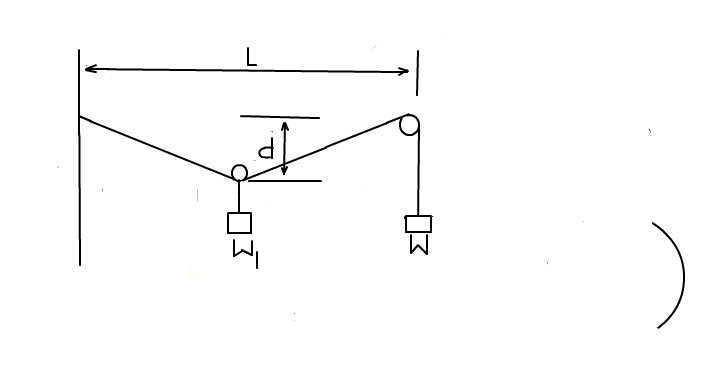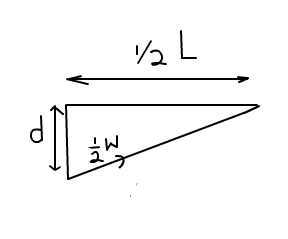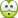# Two weights and two pulleys## Homework Statement

A pulley to which is attached w1 rides on a wire that is attached to a support at the left and that passes over a pulley on the right to weight w.the horizontal distance between the left support and the pulley is L.Express the sag d at the center in terms of w,w1 and L.
The answer is d = 1/2L/√(2w/w1)^2-1.Please help. how do you arrive at the equation for the sag d. sag is the sink or downward bulge.
-

## The Attempt at a Solution

Last edited:

BvU
Homework Helper
Hello Leon,The answer to your question is: make a drawing and identify all the forces that play a role. Then proceed with working out the free body diagrams of the relevant parts -- that are in a steady state, so they must be in equilibrium. In yout list of relevant equationsyou should have relationships that you can apply to that equilibrium state. Post an attempt at solution, even if you get stuck (it is one of the conditions to get help in PF, see the guidelines)

•leonkhasi
i understand that w=1/2w1 is it not?

No they are not necessarily the same, that's why the two weights are differentiated in the answer. BvU is requesting you to upload your attempt at solving the question, please do that so that we may help you•leonkhasi
ouch i'm stuck.BvU
Homework Helper
i understand that w=1/2w1 is it not?
You can check for yourself by considering the limit case: For d >> L it's somewhat true, but the smaller d is, the less it's true: Why should it be true for d ##\rightarrow## 0 ?

BvU
Homework Helper
Good picture. What forces work on W1 ? What forces work on W ? what force is in common ?

[for d →0 i must say w is maximum.or i must say w1 is zero.i'm just guessing how to arrive at the solution but i think its nowhere at all close to the solutionHow about draw some forces? In particular, tension will be useful here.

ehild
Homework Helper
i understand that w=1/2w1 is it not?
No, but the moving pulley might be at the middle.

is d=tanθ⋅L/2? how do i determine how θ changes when i do not know basedwhich of the weights are heavier?

BvU
Homework Helper
W is heavier than W1
##1\over 2##W is not right for the tension in the wire.

•leonkhasi
ehild
Homework Helper
is d=tanθ⋅L/2?
Yes,

Both weights are in equilibrium. The forces the string exerts on them is the tension in the string. Draw the force vectors both at w and w1. They need to add up to zero.

•leonkhasi
do we have to show the equilibrium of the forces at the point at the center ?

Hello there
is w1.g=2t1cosθ? and wat about should t2=w=t1? where t1 and t2 are tensions and should t1=t2 for tension tobe same in the system since force is transmitted on the same rope.

Last edited:
ehild
Homework Helper
Hello there
is w1.g=2t1cosθ? and wat about should t2=w=t1? where t1 and t2 are tensions and should t1=t2 for tension tobe same in the system since force is transmitted on the same rope.
Yes, t1=t2=w. If theta is the angle the string makes with the horizontal then w1.g=2t1cosθ is not true.

i assume θ is the angle between the sag d and the hypotenuse..

ehild
Homework Helper
i assume θ is the angle between the sag d and the hypotenuse..
Then your equation d=tanθ⋅L/2 is not true . (Post #12)

•leonkhasi
if i assume the angle θ like i mention then d=L/2/tan θ yes..but i guess i wont arrive at the solution so i must take θ as angle between hypotenuse and horizontal and d=tanθ.L/2. am i right?

ehild
Homework Helper
if i assume the angle θ like i mention then d=L/2/tan θ yes..but i guess i wont arrive at the solution so i must take θ as angle between hypotenuse and horizontal and d=tanθ.L/2. am i right?
You can use any angle, but do it consequently.